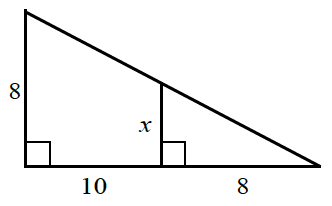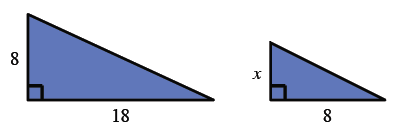### Home > CCAA > Chapter 6 Unit 9 > Lesson CC3: 6.2.6 > Problem6-107

6-107.

Examine the diagram below. The smaller triangle is similar to the larger triangle. Write and solve a proportion to find $x$. It may be helpful to draw the two triangles separately.Draw two separate triangles. The larger triangle has base $10+8=18$.$\frac{x}{8}=\frac{8}{18}$